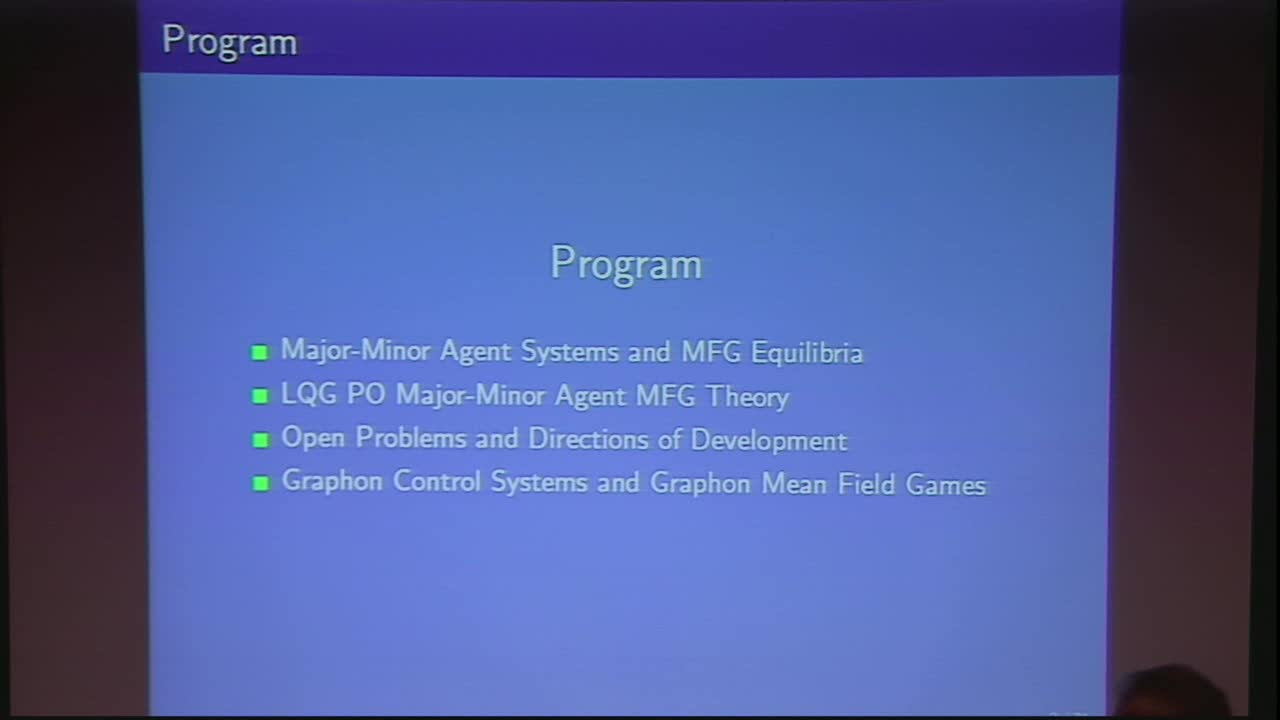## Mean Field Games on Infinite Networks and the Graphon-MFG Equations

May 11, 2018
IMA
Presenters: Peter Caines

Watch Video

#### Abstract

This work introduces Graphon Mean Field Game (GMFG) theory for the analysis of
non-cooperative dynamical games involving agent modelled as controlled stochastic systems distributed over networks of unbounded size. One component is the profoundly influential new graphon theory of large networks and their infinite limits due to Lovasa'z and coworkers which has already been employed in the centralized control of asymptotically infinite networks of systems [Gao and Caines, CDC 2017]. The second component of the theory is that of asymptotically infinite populations of non-cooperative dynamical agents for which equilibria in the standard formulation are expressed in terms of the Mean Field Game equations of Huang, Malhame and Caines, and Lasry - Lions. The newly defined Graphon Mean Field Game (GMFG) equations significantly generalize the classical MFG PDEs since they describe the interaction of infinite populations distributed over infinite networks. In this talk we present existence and uniquenes results for the solutions of GMFG equations together with an epsilon-Nash theorem for the approximate equilibrium behaviour of large finite populations subject to infinite population GMFG feedback control.